Next: Observables Up: Fundamental Concepts Previous: Outer Product

# Eigenvalues and Eigenvectors

In general, the ket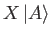is not a constant multiple of the ket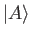. However, there are some special kets known as the eigenkets of operator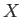. These are denoted(1.45)

and have the property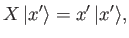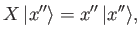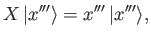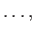(1.46)

where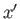,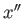,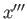,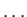are complex numbers called eigenvalues. Clearly, applyingto one of its eigenkets yields the same eigenket multiplied by the associated eigenvalue.

Consider the eigenkets and eigenvalues of an Hermitian operator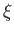. These are denoted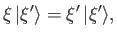(1.47)

where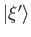is the eigenket associated with the eigenvalue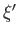. Three important results are readily deduced:
1. The eigenvalues are all real numbers, and the eigenkets corresponding to different eigenvalues are orthogonal. Becauseis Hermitian, the dual equation to Equation (1.47) (for the eigenvalue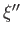) reads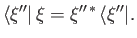(1.48)

If we left-multiply Equation (1.47) by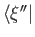, right-multiply the previous equation by, and take the difference, then we obtain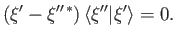(1.49)

Suppose that the eigenvaluesandare the same. It follows from the previous equation that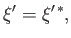(1.50)

where we have used the fact thatis not the null ket. This proves that the eigenvalues are real numbers. Suppose that the eigenvaluesandare different. It follows that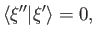(1.51)

which demonstrates that eigenkets corresponding to different eigenvalues are orthogonal.

2. The eigenvalues associated with eigenkets are the same as the eigenvalues associated with eigenbras. An eigenbra ofcorresponding to an eigenvalueis defined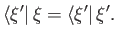(1.52)

3. The dual of any eigenket is an eigenbra belonging to the same eigenvalue, and conversely.Next: Observables Up: Fundamental Concepts Previous: Outer Product
Richard Fitzpatrick 2016-01-22